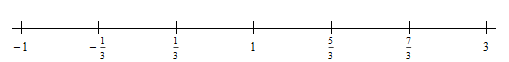Paul's Online Notes
Home / Calculus I / Integrals / Area Problem
Show Mobile Notice Show All Notes Hide All Notes
Mobile Notice
You appear to be on a device with a "narrow" screen width (i.e. you are probably on a mobile phone). Due to the nature of the mathematics on this site it is best views in landscape mode. If your device is not in landscape mode many of the equations will run off the side of your device (should be able to scroll to see them) and some of the menu items will be cut off due to the narrow screen width.

### Section 5.5 : Area Problem

2. Estimate the area of the region between $$g\left( x \right) = 4 - \sqrt {{x^2} + 2}$$ the $$x$$-axis on $$\left[ { - 1,3} \right]$$ using $$n = 6$$ and using,

1. the right end points of the subintervals for the height of the rectangles,
2. the left end points of the subintervals for the height of the rectangles and,
3. the midpoints of the subintervals for the height of the rectangles.

Show All Solutions Hide All Solutions

a The right end points of the subintervals for the height of the rectangles. Show Solution

The widths of each of the subintervals for this problem are,

$\Delta x = \frac{{3 - \left( { - 1} \right)}}{6} = \frac{2}{3}$

We don’t need to actually graph the function to do this problem. It would probably help to have a number line showing subintervals however. Here is that number line.In this case we’re going to be using right end points of each of these subintervals to determine the height of each of the rectangles.

The area between the function and the $$x$$-axis is then approximately,

\begin{align*}{\mbox{Area}} & \approx \frac{2}{3}f\left( { - \frac{1}{3}} \right) + \frac{2}{3}f\left( {\frac{1}{3}} \right) + \frac{2}{3}f\left( 1 \right) + \frac{2}{3}f\left( {\frac{5}{3}} \right) + \frac{2}{3}f\left( {\frac{7}{3}} \right) + \frac{2}{3}f\left( 3 \right)\\ & = \frac{2}{3}\left( {4 - \frac{{\sqrt {19} }}{3}} \right) + \frac{2}{3}\left( {4 - \frac{{\sqrt {19} }}{3}} \right) + \frac{2}{3}\left( {4 - \sqrt 3 } \right) + \frac{2}{3}\left( {4 - \frac{{\sqrt {43} }}{3}} \right) + \frac{2}{3}\left( {4 - \frac{{\sqrt {67} }}{3}} \right) + \frac{2}{3}\left( {4 - \sqrt {11} } \right)\\ & = \require{bbox} \bbox[2pt,border:1px solid black]{{7.420752}}\end{align*}

b The left end points of the subintervals for the height of the rectangles. Show Solution

As we found in the previous part the widths of each of the subintervals are $$\Delta x = \frac{2}{3}$$.

Here is a copy of the number line showing the subintervals to help with the problem.In this case we’re going to be using left end points of each of these subintervals to determine the height of each of the rectangles.

The area between the function and the $$x$$-axis is then approximately,

\begin{align*}{\mbox{Area}} & \approx \frac{2}{3}f\left( { - 1} \right) + \frac{2}{3}f\left( { - \frac{1}{3}} \right) + \frac{2}{3}f\left( {\frac{1}{3}} \right) + \frac{2}{3}f\left( 1 \right) + \frac{2}{3}f\left( {\frac{5}{3}} \right) + \frac{2}{3}f\left( {\frac{7}{3}} \right)\\ & = \frac{2}{3}\left( {4 - \sqrt 3 } \right) + \frac{2}{3}\left( {4 - \frac{{\sqrt {19} }}{3}} \right) + \frac{2}{3}\left( {4 - \frac{{\sqrt {19} }}{3}} \right) + \frac{2}{3}\left( {4 - \sqrt 3 } \right) + \frac{2}{3}\left( {4 - \frac{{\sqrt {43} }}{3}} \right) + \frac{2}{3}\left( {4 - \frac{{\sqrt {67} }}{3}} \right)\\ & = \require{bbox} \bbox[2pt,border:1px solid black]{{8.477135}}\end{align*}

c The midpoints of the subintervals for the height of the rectangles. Show Solution

As we found in the first part the widths of each of the subintervals are $$\Delta x = \frac{2}{3}$$.

Here is a copy of the number line showing the subintervals to help with the problem.In this case we’re going to be using midpoints of each of these subintervals to determine the height of each of the rectangles.

The area between the function and the $$x$$-axis is then approximately,

\begin{align*}{\mbox{Area}} & \approx \frac{2}{3}f\left( { - \frac{2}{3}} \right) + \frac{2}{3}f\left( 0 \right) + \frac{2}{3}f\left( {\frac{2}{3}} \right) + \frac{2}{3}f\left( {\frac{4}{3}} \right) + \frac{2}{3}f\left( 2 \right) + \frac{2}{3}f\left( {\frac{8}{3}} \right)\\ & = \frac{2}{3}\left( {4 - \frac{{\sqrt {22} }}{3}} \right) + \frac{2}{3}\left( {4 - \sqrt 2 } \right) + \frac{2}{3}\left( {4 - \frac{{\sqrt {22} }}{3}} \right) + \frac{2}{3}\left( {4 - \frac{{\sqrt {34} }}{3}} \right) + \frac{2}{3}\left( {4 - \sqrt 6 } \right) + \frac{2}{3}\left( {4 - \frac{{\sqrt {82} }}{3}} \right)\\ & = \require{bbox} \bbox[2pt,border:1px solid black]{{8.031494}}\end{align*}